• ## 关系运算

万次阅读 多人点赞 2019-03-21 14:34:02
关系运算的运算对象是关系，运算结果亦是关系，关系代数的运算符包括两类：传统的集合运算和专门的关系运算两类。 传统的集合运算是从关系的水平方向，即行的角度来进行 而专门的关系代数不仅涉及行，还涉及列。 ...
关系代数是一种抽象的查询语言，它用对关系的运算来表达查询。

关系运算的运算对象是关系，运算结果亦是关系，关系代数的运算符包括两类：传统的集合运算和专门的关系运算两类。 传统的集合运算是从关系的水平方向，即行的角度来进行 而专门的关系代数不仅涉及行，还涉及列。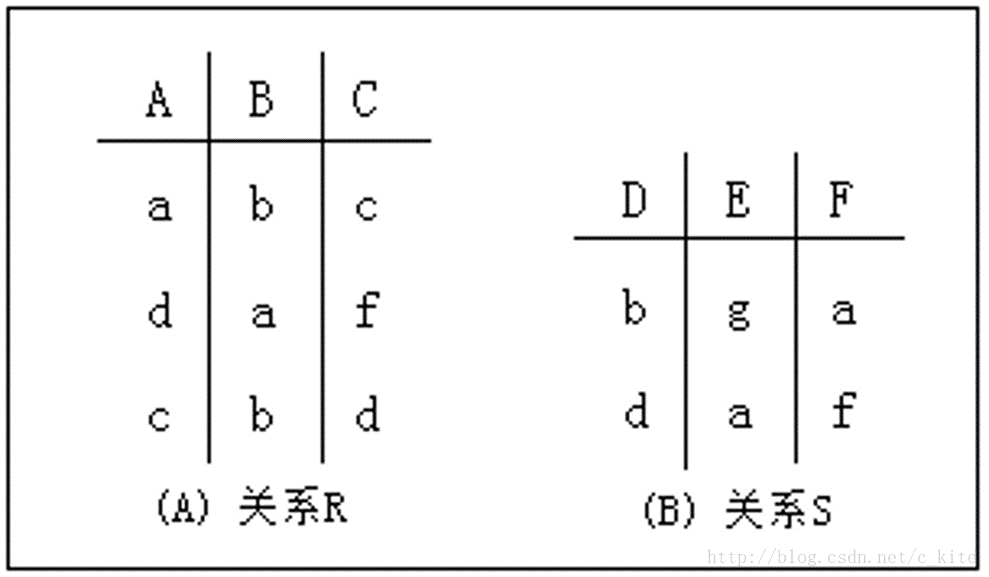传统的集合运算
传统的集合运算是二目运算，包括并，差，交，笛卡尔积4种运算。
并（Union，表示为U）：两个表或集合的联合。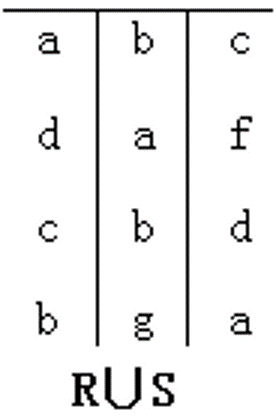例：R1=（A，B），R2=（B，C，D）。 则R1UR2=（A，B，C，D）。 注：U集里不包含重复的属性。
差（Difference，表示位-）：两个表或集合的区别。
例：R1=（A，B），R2=（B，C，D）。
则R1-R2=（A）。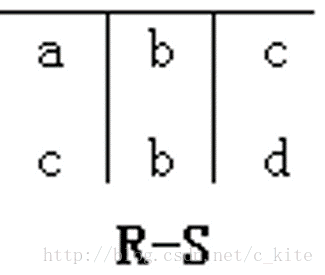注：-集里的元素个数不能大于初始。
交（Intersection，∩）：两个及以上的集合或表中具有相同属性的集合。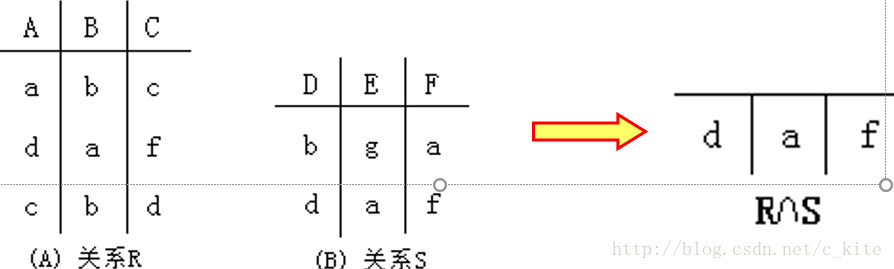笛卡儿积（Product，表示为X）：两个表或集合的组合个数。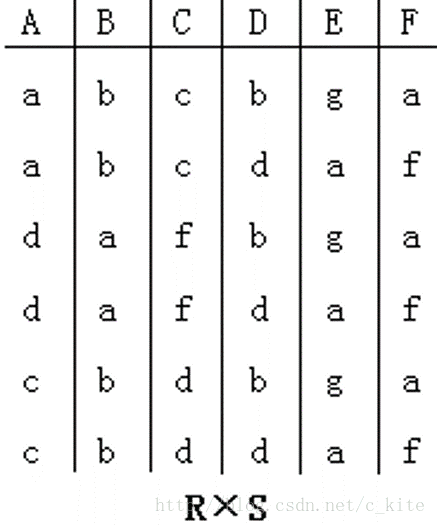例1：R1=（A，B），R2=（B，C，D）。
注：R1xR2集的元素个数为R1的元素个数乘R2的元素个数；
专门的关系运算
投影（Project，表示为π）：从表中抽取特定的列值。
表达式：πM（R）={ t(M) |t∈R }.
释义：R表示一个关系表；
T表示R中的一条横向的记录；
M表示T中的M列的交叉属性值；
πM（R）={ t(M) |t∈R }表示在关系表R中T行M列的一个属性值；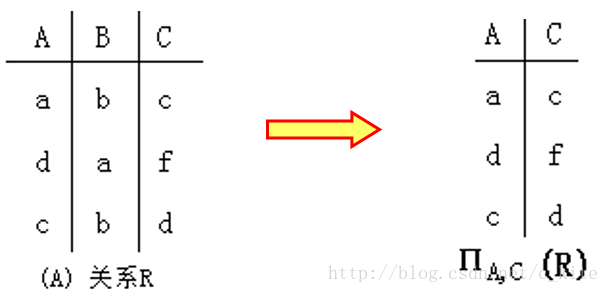选择（Select，表示为σ–Sigma）:从表中选取与给定条件相符的行。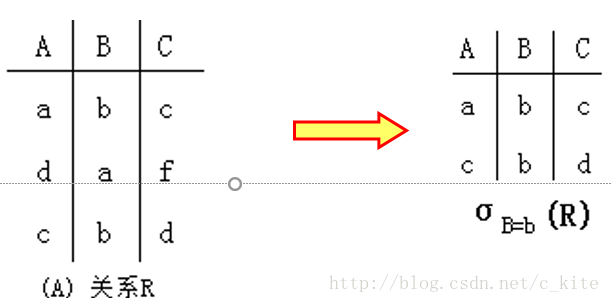表达式：σA=a（R）={ t(A)=a |t∈R }.
A表示R表中的一个字段或属性类型；
t∈R表示R表中的一条记录；
t(A)=a表示记录t中A属性的具体值等于a;
σA=a（R）={ t(A)=a |t∈R }表示在R表中选择A=a的一条记录；
联接（join,表示为▷◁）：通过共同属性连接两个表。
连接运算中有两种最为常见的连接。一种是等值连接还有一种为自然连接。等值连接为从R和S的笛卡尔积中选取那些R和S的公共属性值都相等的那些元组，进行等值连接。 自然连接是一种特殊的等值连接，在等值连接的基础上去掉那些R和S都有的公共属性列，就是自然连接。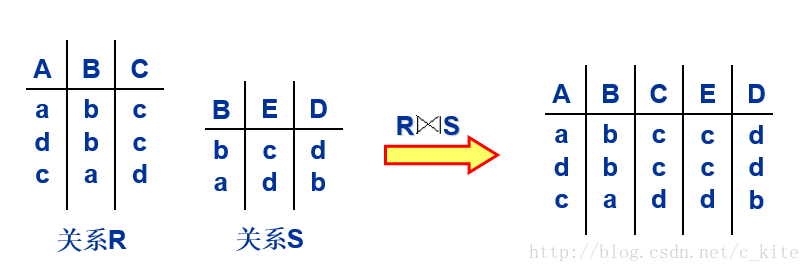表现在数据库中简单来说是通过字段值相同的条件下，将两个表中的记录连接在一起。
除（Division，表水为÷）：除运算需要满足两个条件：表R和表S的属性集合要有相同性；R÷S的商是R和S非相同属性集合的一个投影的子集，该子集和S的笛卡尔积必须包含在R中。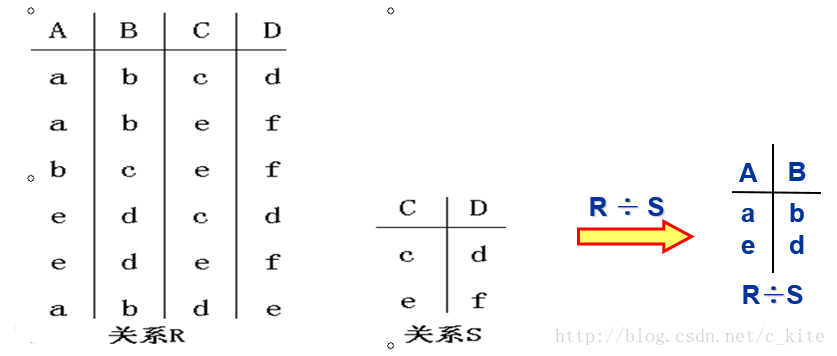展开全文关系模型 关系代数
• 关于关系运算 大家对算术运算比较熟悉，比如：3 + 4 得 7。 关系运算也是一种运算，比如：3 > 4 得 0。 关系运算是比较特殊的运算，它的具体工作是减法！ 3 > 4 实际是求 3 - 4 得到一个负数， 由此可知：3 不...
• 谭浩强老师《C程序设计》第四章第一题。...2.关系运算就是比较运算，将两个数值进行比较，判断其比较结果是否符合给定的条件。 3.逻辑运算指两个条件进行运算，有逻辑与、逻辑或、逻辑非三种。 ...
谭浩强老师《C程序设计》第四章第一题。学习辅导里没有答案，整理一下方便记忆。
1.算术运算就是指加减乘除和整数的模运算（即取余数运算）。
2.关系运算就是比较运算，将两个数值进行比较，判断其比较结果是否符合给定的条件。
3.逻辑运算指两个条件进行运算，有逻辑与、逻辑或、逻辑非三种。
展开全文什么是算数运算 什么是逻辑运算
• 算术运算 算术运算:用于各类数值运算.包括加(+)、减(-)、乘(*)、除(/)、求余(或称模运算,%)、自增(++)、自减(–)共七种. 算术运算简称运算。 指按照规定的法则和顺序对式题或算式进行运算，并求出结果的过程。包括...
什么是算术运算？什么是关系运算？什么是逻辑运算？
【答案解析】
算术运算：
算术运算即“四则运算”，是加法、减法、乘法、除法、乘方、开方等几种运算的统称。  其中加减为一级运算，乘除为二级运算，乘方、开方为三级运算。在一道算式中，如果有多级运算存在，则应先进行高级运算，再进行低一级的运算。  C语言中的算熟运算符包括：+、-、*、/、++、--、% 等种类。  如果只存在同级运算；则从左至右的顺序进行；如果算式中有括号，则应先算括号里边，再按上述规则进行计算。
示例：$(1 + 1)^{2} * 4+5 * 3$
解析：
先进行括号内运算1+1，然后进行乘方运算得到结果4.接下来与4相乘，得到结果16因为乘法优先级大于加法，因此先进行5*3，得到结果15最终相加得到结果31
结果：31
关系运算：
关系的基本运算有两类：一类是传统的集合运算（并、差、交等），另一类是专门的关系运算（选择、投影、连接、除法、外连接等），而在C语言中，关系运算通常被认为是比较运算，将两个数值进行比较，判断比较结果是否符合给定的条件。  常见的关系运算符包括：<、<=、>、>=、==、!= 等种类。  其中，前4种关系运算符(<、<=、>、>= )的优先级别相同，后2种(==、!=)也相同。而前4种高于后2种。  例如, > 优先于 == 。而 > 与 < 优先级相同。 并且，关系运算符的优先级低于算术运算符，关系运算符的优先级高于赋值运算符(=)。
逻辑运算：
在逻辑代数中，有与、或、非三种基本逻辑运算。表示逻辑运算的方法有多种，如语句描述、逻辑代数式、真值表、卡诺图等。而在C语言中，逻辑运算通常用于使用逻辑运算符将关系表达式或其它逻辑量连接起来组成逻辑表达式用来测试真假值。  常见的逻辑运算符包括：&&、||、! 等种类  &&： 与是双目运算符，要求有两个运算对象，表示两个运算对象都成立，则结果为真，否则结果为假。
例如：(a<b) && (x>y)，表示(a<b)和(x>y)同时成立则为真。
||：是双目运算符，要求有两个运算对象，表示两个运算对象只要任意一个成立，则结果为真，否则结果为假。  例如：(a<b) && (x>y)，表示(a<b)和(x>y)两个对象中任意一个成立则结果为真。  !：是单目运算符，只要求有一个运算对象，表示取运算对象反义，运算对象为真则结果为假，运算对象结果为假则结果为真。  例如：!(a>b)，表示(a>b)成立时结果为假，不成立时结果为真。  若在一个逻辑表达式中包含多个逻辑运算符，则优先次序为： ! > && > ||。当然若一个逻辑表达式中包含括号括起来的子逻辑，则优先括号内的子逻辑判断。
示例：
(1>2)||(2>3)&&(4>3) 结果为0 !(1>2)||(2>3)&&(4>3)结果为1  注：&&优先级大于||，((2>3)&&(4>3))无法同时成立，则结果为假，然后与(1>2)结果进行逻辑或运算，两者都为假因此第一次结果为假。 而第二次!优先级最高，先对(1>2)的结果取逻辑非，得到结果为真，因此结果为真。 
展开全文运算 算数运算 逻辑运算
• 关系运算的机理有什么用 我们学习关系运算的机理，对我们理解数据库查询操作非常重要 所以我们进行关系操作时很大程度上需要明白关系操作以及关系之间的逻辑 在我们进行数据库查询操作时，如何规范的使用数据库语言...
• 一、关系运算 、 二、关系运算示例 、 三、关系运算性质


文章目录
一、关系幂运算二、关系幂运算示例三、关系幂运算性质

一、关系幂运算

关系

R

R

的

n

n

次幂定义 :

R

⊆

A

×

A

,

n

∈

N

R \subseteq A \times A , n \in N

{

R

0

=

I

A

R

n

+

1

=

R

n

∘

R

(

n

≥

0

)

\begin{cases} R^0 = I_A & \\ R^{n +1} = R^n \circ R & ( n \geq 0 ) \end{cases}

关系

R

R

是 集合

A

A

上的 二元关系 ,

R

R

的

0

0

次幂

R

0

R^0

是恒等关系

I

A

I_A

, 关系

R

R

的

n

+

1

n + 1

次幂等于

R

n

+

1

=

R

n

∘

R

R^{n + 1} = R^n \circ R

其中

n

≥

0

n \geq 0

;

R

1

=

R

0

∘

R

=

R

R^1 = R^0 \circ R = R

, 恒等关系与 关系

R

R

逆序合成 , 结果还是关系

R

R

, 这个关系

R

R

可以是任意关系 ;
恒等关系就是 集合

A

A

中每个元素自己跟自己有关系 ;
关系

R

R

幂运算结果

R

n

R^n

关系 也是集合

A

A

上的二元关系 , 因此有

R

n

⊆

A

×

A

R^n \subseteq A \times A

关系

R

R

的

n

n

次幂 , 就是

n

n

个

R

R

关系逆序合成 :

R

n

=

R

∘

R

∘

⋯

∘

R

⏟

n

个

R

逆

序

合

成

R^n = \begin{matrix} \underbrace{ R \circ R \circ \cdots \circ R } \\ n 个 R 逆序合成 \end{matrix}

二、关系幂运算示例

集合

A

=

{

a

,

b

,

c

}

A = \{ a, b, c \}

关系

R

R

是 集合

A

A

上的二元关系 ,

R

⊆

A

×

A

R \subseteq A \times A

,

R

=

{

<

a

,

b

>

,

<

b

,

a

>

,

<

a

,

c

>

}

R = \{ <a,b> , <b,a> , <a, c> \}

关系

R

R

的 幂集个数 :

A

A

是有限集 ,

A

A

上的有序对个数是

3

×

3

=

9

3 \times 3 = 9

个 ,

A

A

上的二元关系个数 , 即有序对集合的幂集个数 , 是

2

3

×

3

=

512

2^{3\times 3} =512

个 ;

关系

R

R

的

0

0

次幂 :

R

0

=

I

A

R^0 = I_A

,

R

R

关系的

0

0

次幂是恒等关系 , 关系图是每个顶点都有环 , 顶点之间没有关系 ;关系

R

R

的

1

1

次幂 :

R

1

=

R

0

∘

R

=

R

R^1 = R^0 \circ R = R

, 恒等关系

I

A

I_A

与任何关系逆序合成 , 结果还是那个关系 ;关系

R

R

的

2

2

次幂 :

R

2

=

R

0

∘

R

=

R

∘

R

=

{

<

a

,

b

>

,

<

b

,

a

>

,

<

a

,

c

>

}

∘

{

<

a

,

b

>

,

<

b

,

a

>

,

<

a

,

c

>

}

=

{

<

a

,

a

>

,

<

b

,

b

>

,

<

b

,

c

>

}

\begin{array}{lcl}R^2 & = & R^0 \circ R \\\\ &=& R \circ R \\\\ &=& \{ <a,b> , <b,a> , <a, c> \} \circ \{ <a,b> , <b,a> , <a, c> \} \\\\ &=& \{ <a,a>, <b, b> , <b,c> \}\end{array}

注意上述

∘

\circ

运算时逆序合成 , 从后面的关系中合成前面的关系 ;关系

R

R

的

3

3

次幂 : 与

R

1

R_1

相同

R

3

=

R

1

∘

R

=

{

<

a

,

a

>

,

<

b

,

b

>

,

<

b

,

c

>

}

∘

{

<

a

,

b

>

,

<

b

,

a

>

,

<

a

,

c

>

}

=

{

<

a

,

b

>

,

<

a

,

c

>

,

<

b

,

a

>

}

=

R

1

\begin{array}{lcl}R^3 & = & R^1 \circ R \\\\ &=& \{ <a,a>, <b, b> , <b,c> \} \circ \{ <a,b> , <b,a> , <a, c> \} \\\\ &=& \{ <a,b>, <a, c> , <b,a> \} \\\\ &=& R^1 \end{array}关系

R

R

的

4

4

次幂 : 与

R

2

R_2

相同

关系

R

R

的

5

5

次幂 : 与

R

1

R_1

相同

关系

R

R

的

2

k

2k

偶数次幂 (

k

=

1

,

2

,

⋯

k=1,2, \cdots

) : 与

R

2

R_2

相同

关系

R

R

的

2

k

+

1

2k + 1

奇数次幂 (

k

=

0

,

1

,

2

,

⋯

k=0,1,2, \cdots

) : 与

R

1

R_1

相同

三、关系幂运算性质

关系幂运算性质 :
关系

R

R

是 集合

A

A

上的关系 ,

R

⊆

A

×

A

R \subseteq A \times A

,

m

,

n

m,n

是自然数 ,

m

,

n

∈

N

m,n \in N

; 关系幂运算有以下两个性质 :

R

m

∘

R

n

=

R

m

+

n

R^m \circ R^n = R^{m + n}

(

R

m

)

n

=

R

m

n

(R^m ) ^n = R^{m n}


展开全文• 关系代数 关系运算 讲解 课程 关系代数 关系运算 讲解 课程
• 数据库 关系运算 ppt 讲述了数据库关系运算的基本概念。数据库
• package 比较运算和关系运算一样叫法不一样 import ( “fmt” ) func main(){ //关系运算符（比较运算符） //关系运算符的结果都是bool型，也就是要么是true，要么是false //关系表达式经常用在if结构的条件中或循环...
• ## 数据库关系运算——除运算

万次阅读 多人点赞 2018-07-02 22:29:05
书上给“除运算”的定义是： 设关系R除以关系S的结果为关系T，则T包含所有在R但不在S中的属性及其值，且T的元组与S的元组的所有组合都在R中。 我对此不是很理解。 直到看到这样的解读，方才恍然大悟： ...
• 专门的关系运算并（Union）R和S 具有相同的目n（即两个关系都有n个属性） 相应的属性取自同一个域R∪S 仍为n目关系，由属于R或属于S的元组组成 R∪S = { t|t  R∨t S } 差（Difference）R和S 具有相同的目n ...数据库 关系代数 union 属性
• 小插曲：小编在学习计算机二级的时候，苦于关系代数的关系运算理解，在网上百度很多的资源，大都是概念，而且是文本形式呈现的。个人喜好图文形式的理解，因此将做的比较好的ppt做成帖子供大家参考，如有错误，请...计算机二级 关系代数
• ## 数据库关系运算

万次阅读 多人点赞 2017-03-16 19:18:14
本文就是来讲解数据库中的各种关系运算的！本文不做数学概念的深入，只要理解相关的概念即可！ 为什么我们要学习数据库关系运算？学习和理解关系运算的机理，对于理解关系数据库中的数据查询机制有十分重要的意义。...数据库
• 关系代数中，个人觉得比较容易搞混的有这两个概念：传统集合运算和专门关系运算； 传统集合运算：并(∪)、交(∩)、差(—)、笛卡尔积(×)； 专门关系运算：选择(σ)、投影(Π)、连接(∞)、除(÷)； 传统的集合运算将...数据库
• ## 数据库原理之关系数据库关系运算

万次阅读 多人点赞 2018-12-06 10:10:31
关系数据库关系运算 选择 投影 链接 除运算 选择运算 选择运算是从关系R中选取使逻辑表达式F为 真的元组，是从行的角度进行的运算 投影运算 投影操作主要是从列的角度进行运算，但投影之后...
• MATLAB关系运算 MATLAB关系运算符对数组的元素进行设置，将元素设置为逻辑1（真）的关系是真实的和逻辑0（假），它是两个阵列，可以返回一个相同大小的逻辑阵列间的比较。 MATLAB关系运算符标和非标量数据上也能...
• ## 关系运算优化

千次阅读 2015-10-07 19:18:27
关系运算的安全约束和等价性 在数据库技术中，不产生无限关系和无穷验证的运算称为安全运算，相应的表达式称为安全表达式，所采取的措施称为安全约束。 并、差、笛尔卡积、投影和选择是关系代数最基本的操作，并...数据库
• 目的 1.学习关系代数基本操作，包括并、差、积、投影、选择、更名。 2.学习关系代数扩展操作，包括交，theta-连接，自然...广义笛卡尔积：两个集合中拼接元组的所有可能的集合，链接运算的基础。 选择：给一个条件，选数据库
• 查询语言 用户用来从数据库中请求获取信息的语言 “纯”查询语言（奠定了...六个基本运算 select 选择 project 投影 union 并 set difference 差（合集差） cartesian product 笛卡尔积 rename 更名（重命...
• ## 关系代数运算

千次阅读 2019-02-01 15:55:18
关系运算的参与对象是关系，运算后结果也是关系。在数据库中，关系就是一张张的表。在离散数学中，关系是做为一个个的集合来进行运算的。 代数运算的分类： 传统的集合运算：并、差、交、笛卡儿积、笛卡儿积的逆...
• 今天把逻辑运算和关系运算的小节看了。 比较有帮助的，在于长路运算和短路运算。 本来我在C当中，只学习了&&、||这样的与、或运算。 但是java当中，有短路运算，以及非短路运算。 例如： (x=0时，则结果必为...
• ## 关系代数运算之连接运算

万次阅读 多人点赞 2019-04-20 18:28:17
1.关于连接的理解：连接也称为θ连接，关系R与关系S的连接运算是从两个关系的笛卡尔积中选取属性间满足一定条件的元组形成一个新的连接。这个条件为θ(比较运算符，如>、<、=)。 2.自然连接：是一种特殊的...连接
• 关系代数是一种抽象的查询语言，它用对关系的运算来表达查询，其运算对象是关系、运算结果亦为关系，关系代数的运算符有...传统的集合运算是从关系的“水平”方向即行的角度进行，专门的关系运算不仅涉及行而且涉及列。数据库系统原理 笛卡儿积
•matlab
• 1. 关系运算 关系代数的基本运算主要有并、交、差、笛卡尔积、选择、投影、连接和除法运算。 （1）并。计算两个关系在集合理论上的并集，即给出关系R和S（两者有相同元/列数），R∪S的元组包括R和S所有元组的集合，...
• 除法运算的定义： 这个概念的描述的非常抽象，刚开始学习的同学完全不知所云。这里通过一个实例来说明除法运算的求解过程： 设有关系R、S 如图所示，求R÷S 的结果： 求解步骤过程： 第一步：找出关系R和关系S中...数据库 sql 计算
•笛卡尔积 连接...